Courses

# Test: Viscous Flow of Incompressible Fluids, Flow Losses Level - 1

## 20 Questions MCQ Test Engineering Mechanics | Test: Viscous Flow of Incompressible Fluids, Flow Losses Level - 1

Description
This mock test of Test: Viscous Flow of Incompressible Fluids, Flow Losses Level - 1 for Civil Engineering (CE) helps you for every Civil Engineering (CE) entrance exam. This contains 20 Multiple Choice Questions for Civil Engineering (CE) Test: Viscous Flow of Incompressible Fluids, Flow Losses Level - 1 (mcq) to study with solutions a complete question bank. The solved questions answers in this Test: Viscous Flow of Incompressible Fluids, Flow Losses Level - 1 quiz give you a good mix of easy questions and tough questions. Civil Engineering (CE) students definitely take this Test: Viscous Flow of Incompressible Fluids, Flow Losses Level - 1 exercise for a better result in the exam. You can find other Test: Viscous Flow of Incompressible Fluids, Flow Losses Level - 1 extra questions, long questions & short questions for Civil Engineering (CE) on EduRev as well by searching above.
QUESTION: 1

### The pressure drop for a flow with relatively low Reynolds number through a 600 mm diameter, 30 m long pipeline is 70 kPa. What is the wall shear stress?

Solution: For flow through pipes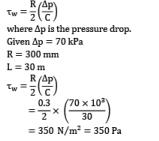QUESTION: 2

### For a pipe of circular cross section, assuming Darcy Weisbach equation holds and free stream velocity is constant

Solution: Darcy Weisbach equation states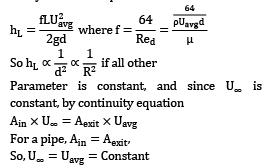QUESTION: 3

### Find the loss of head due to friction in a smooth pipe of rectangular cross section of 1 m × 0.5 m and 10 m long. Velocity of flow is 10 m⁄s and the friction coefficient is 0.01962.

Solution: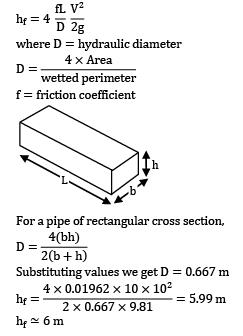[Note: Darcy friction factor = 4 × friction coefficient]

QUESTION: 4

The purpose of surge tank in a pipe line is to

Solution: Surge tank is used to decrease the pressure fluctuation in the pipe which causes water hammer.

QUESTION: 5

Head loss in turbulent flow in a pipe

Solution: For turbulent flows head loss is proportional to V2 (approximately).

QUESTION: 6

Oil at 20°C (ρ = 888 kg⁄m3 and μ = 0.800 kg/m ∙ s) is flowing steadily through a 5 cm diameter, 40 m long pipe. The pressure at the pipe inlet and outlet are measured to be 745 and 97 kPa, respectively. Determine the flow rate of oil (in L/s) through the pipe assuming the pipe is horizontal.

Solution:

ρ = 888 kg⁄m3 μ = 0.8 kg⁄m d = 5 cm or r = 2.5 cm

L = 40 m Hagen Poiseuille formula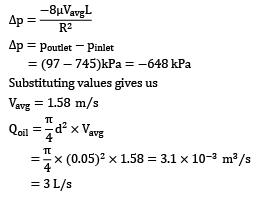QUESTION: 7

Water at 25°C is flowing through a 1.0 km long GI pipe of 200 mm diameter at the rate of 0.07 m3⁄s. If value of Darcy friction factor for this pipe is 0.02 and density of water is 1000 kg/m3, the pumping power (in kW) required to maintain the flow is

Solution: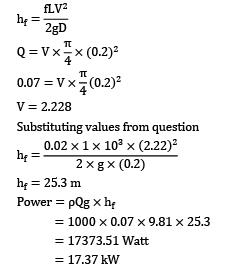QUESTION: 8

Pipes of lengths l1 , l2 etc. and diameters d1 , d2 , etc., are connected in series. A single pipe of length l1 + l2 + l3 +……having same pressure loss as all pipes in series will have diameter equal to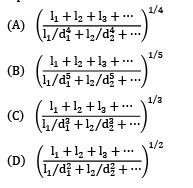Solution: For pipes in series we know hfequivalent = hf1 + hf2 … . . hfm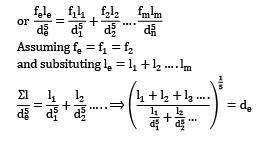QUESTION: 9

The velocity distribution for a fully-developed laminar flow in a pipe is given by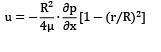Determine the radial distance from the pipe axis at which the velocity equals the average velocity.

Solution: The given velocity profile is the velocity profile of fully developed laminar flow in a pipe. We know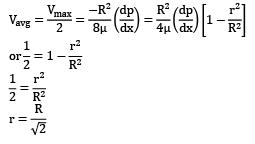QUESTION: 10

Heavy fuel oil flows from A to B through a 100 m horizontal steel pipe of 150 mm diameter. The pressure at A is 1.08 MPa and at B is 0.95 MPa. The kinematic viscosity is 412.5 × 10−6 m2⁄s, and the relative density of the oil is 0.918. What is the flow rate in m3⁄s?

Solution: In laminar flow through pipe, we know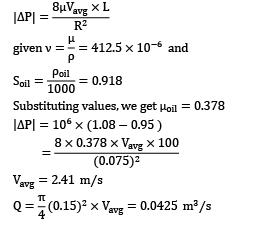QUESTION: 11

In the case of fully developed flow through tubes

Solution: f = 4Cf

QUESTION: 12

The Reynolds number for flow of oil through a 5 cm diameter pipe is 1700. The kinematic viscosity, v = 1.02 × 10−6 m2⁄s. What is the velocity at a point 0.625 cm away from the wall?

Solution: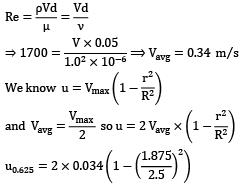Note: r is taken from the center and not from the wall, so for a section 0.625 cm from wall has r = R − 0.625 = 1.875 cm] u0.625 = 0.0297 = 0.03 m⁄s

QUESTION: 13

Three identical pipes of length l, diameter d and friction factor f are connected in parallel between two reservoirs. What is the size of a pipe of length l and of the same friction factor f equivalent to the above pipe?

Solution: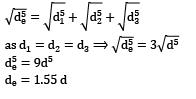QUESTION: 14

The extent of pressure produced due to water hammer depends on

Solution: The pressure produced due to water hammer depends on the mass of the water moving in the pipe (which is turn depends on the length of the pipe), the velocity of the moving water and how slowly or rapidly the valve is closed.

QUESTION: 15

The head loss in a sudden expansion from 8 cm diameter pipe to 16 cm diameter pipe in terms of velocity V1 in the smaller pipes is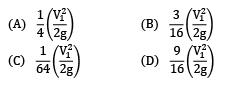Solution: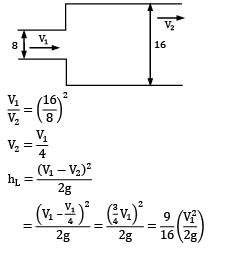QUESTION: 16

Flow takes place at Reynolds number of 1500 in two different pipes with relative roughness of 0.001 and 0.002. The friction factor

Solution: Darcy friction factor depends only on the Reynolds number of the flow. The critical value of Reynolds number (at which laminar to turbulent transition occurs) depends on surface roughness. But the friction factor has no dependence on the surface finish of the pipe.

QUESTION: 17

For laminar flow through a round pipe, the shear stress

Solution: Shear stress is directly proportional to the radial distance from center and opposite to the direction of flow velocity.

QUESTION: 18

Laminar flow between closely spaced parallel, stationary plates is governed by the consideration of which one of the following pair of forces?

Solution: For laminar flow, viscous forces must come into picture, and viscous effects leads to head loss, hence a pressure force is required to maintain the flow. If the plates are horizontal, the gravitational effects can be ignored.

QUESTION: 19

Which one of the following statements is not correct in the context of laminar flow through a pipeline?

Solution: A die injected into the flow stream will form a streak line and if the flow is steady then it will be equivalent to stream and path line. So the die will disperse.

QUESTION: 20

The center-line velocity in a flow between parallel plates is 3 m⁄s. What is the average flow velocity between the plates if the Reynolds number of the flow is 800?

Solution: For flow between parallel plates, average velocity is given as,

Vavg = 2 / 3Vmax.

Given centerline velocity, Vmax = 3 m/s

∴ Vavg = 2 / 3 × 3 = 2 m/s